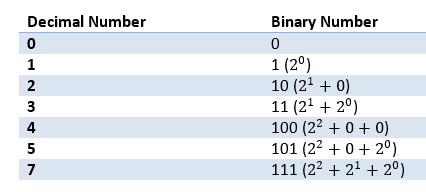## Saturday, May 17, 2014

### PowerShell Bit Manipulation and Network Subnets

Many IT professionals get scared when the topic of binary numbers and bit manipulation come up. This is not complex, once understood it opens up a whole realm of possibilities. One concrete example where bit manipulation comes in handy is when dealing with subnets. This blog briefly covers bit manipulation and applies the technique to check if a given IP address belong to a Subnet. If you are familiar with bit manipulation skip directly to the code.

# Binary Numbers

Computer understands only ‘1’s and ‘0’s (i.e.) binary. All numeric values in computer are stored as binary numbers.  Once you understand the basics of binary numbers and the binary manipulation you can implement efficient code in some cases. In the decimal system each place of digit goes by powers of ten, while in binary system it goes by powers of two. If you are not familiar read at The Binary System.# Bit Manipulation

In PowerShell there are binary equivalent of logical operations. (e.g.) band is binary-and. In that the binary-and applies the ‘and’ operator for each bit in operand1 with corresponding bit in operand2 to produce the corresponding bit in the result. (e.g.) (4 –band 5) will produce 4. How? In the binary 4 and 5 are represented as ‘100’ and ‘101’ respectively. Logical and of least significant bits will result in zero, second significant will also result in zero, while most significant will result in 1 (because both of them are 1). Thus the result in binary is ‘100’ which is decimal ‘4’.

Another nice operator is the shift operator. Shift left operator shifts the bits by ‘n’ binary digits and adds ‘n’ zeros on the right. (2 –shl 2) will result in 8 (because ‘10’ is shifted by two digits and two zeroes added to the right, resulting in ‘1000’, which is decimal 8)

Likewise binary-not will flip every single bit in a binary number (i.e.) If it is ‘1’ it will turn to ‘0’, if it ‘0’ it will turn to ‘1’.  ((-bnot 4) –band 7) will result in 3. Why? Decimal ‘4’ is equal to ‘...000100’ will result in ‘…111011’. Do a binary-and with 7 to retrieve least 3 significant bits. The result is ‘011’ which is ‘3’. The reason ‘7’ is chosen for binary-and is, it has all three digits set to ‘1’ (i.e.) ‘111’. Just doing a (-bnot 4) will result in ‘-5’, can you figure out why? This will lead to the understanding of how binary form of negative numbers are represented.

There are many other binary operators in PowerShell like shr, bor, bxor.

IP Address is a 32 bit number consists of two parts the network part and the host part. Classless Internet Domain Routing (CIDR) is the way to represent the subnet. CIDR has the network address and the length, which is the number of bits that constitute the network part of the IP Address. (e.g.) 192.168.0.0/16 means ‘198.168.0.0’ is the network address and 16 bits are used to represent the network part. This means the subnet mask is ‘255.255.0.0’.

The network part of any IP address can easily be obtained by doing binary-and (band) of the subnet mask with the IP address. Say the IP address is ‘192.168.0.22’ and the subnet mask ‘255.255.0.0’, doing a binary-and of them will result in 192.168.0.0’.

# Code for CheckSubnet

function checkSubnet ([string]\$cidr, [string]\$ip)
{
\$network, [int]\$subnetlen = \$cidr.Split('/')
\$a = [uint32[]]\$network.split('.')
[uint32] \$unetwork = (\$a -shl 24) + (\$a -shl 16) + (\$a -shl 8) + \$a

\$mask = (-bnot [uint32]0) -shl (32 - \$subnetlen)

\$a = [uint32[]]\$ip.split('.')
[uint32] \$uip = (\$a -shl 24) + (\$a -shl 16) + (\$a -shl 8) + \$a

}

Sample usage:
PS C:\> checkSubnet ‘192.168.240.0/20’ ‘192.168.250.10’
True

PS C:\> checkSubnet ‘192.168.240.0/20’ ‘192.168.150.10’
False

The code is pretty dense and requires explanation.

\$network, [int]\$subnetlen = \$cidr.Split('/')

The above code splits the CIDR into the network part and the length part and stores it in \$network and \$subnetlen respectively. It also converts the length into integer.

\$a = [uint32[]]\$network.split('.')
[uint32] \$unetwork = (\$a -shl 24) + (\$a -shl 16) + (\$a -shl 8) + \$a

Uint32 is an unsigned integer and can store up to 32 bits. Hence this can store the IP address. 32 bits has four bytes. We have the network as a string of four bytes with ‘.’ delimiter between each byte. To convert to uint32, first extract each byte and put it in appropriate place of uint32. Extracting each byte is simple, can be achieved by split operation. The trick is to form the 32 bit number and placing each byte in the appropriate place. To put \$a in least significant byte just add it. To put \$a one byte up, you need to left shift by 8 bits and then add it. (Note: 8 bits corresponds to one byte, which is why you are shifting 8 bits to move one byte up). Likewise you need to shift \$a by 16 bits, \$a by 24 bits. That does the trick!

\$mask = (-bnot [uint32]0) -shl (32 - \$subnetlen)

To calculate the subnet mask, first create a 32 bit number with all ‘1’. This can easily be achieved by taking the binary-not of zero. (-bnot [uint32]0). Zero has to be casted to uint32 because PowerShell defaults to int32, hence results in a negative number. Number of zeroes in the subnet mask is equal to (32 - \$subnetlen). Just do a left shift by this amount to achieve the subnet mask.

\$a = [uint32[]]\$ip.split('.')
[uint32] \$uip = (\$a -shl 24) + (\$a -shl 16) + (\$a -shl 8) + \$a

Above code does the same manipulation for the ip address that was done for the network part. Now you have uint32 version of both network and ip address.